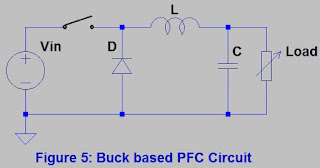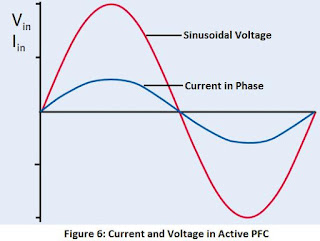# Types of Power Factor Correction

Previous blog explained the "Power Factor". You can find more on this at;
"What is Power Factor and Why is it Important?"

Now let's see types of Power Factor Correction.
There are two types of Power Factor Correction techniques; Passive PFC and Active PFC.
Let’s understand one by one.

### Passive Power Factor Correction

Passive components like inductors and capacitors are used in Passive PFC to correct reduced power factors. These capacitors and inductors are tuned to the line frequency in a low pass or band pass configuration. The Passive PFC normally uses a simple line-frequency LC filter to extend the current conduction angle and decrease the THD of the input current of the diode-capacitor rectifier. This type of power factor correction method corrects the power factor in between 0.7 to 0.85.
Physical size and weight of these filters at mains frequency makes them nasty, particularly when one considers that the circuitry size and form factor should be small.

Types of Passive Power Factor Correction;

#### Capacitor input filter

This type is also called as the π filter; it removes unwanted frequencies from a signal. This filter reduces the harmonic content of a current waveform by making sure that the cut-off frequency of the filter is just above the fundamental frequency. As a result, the best possible reduction of harmonics can be achieved. Below figure shows a π filter;pi Filter Circuit

#### Valley Fill PFC

One more way to achieve power factor improvement up to 0.85 using simple, low cost circuitry shown below;Valley Fill PFC Circuit
This power-factor corrector can be used in low-power applications, where a high effective ripple voltage on DC output can be tolerated. It is frequently used in electronic ballast applications. The circuit contains two capacitors and three diodes. The two capacitors are charged in series around the line peak to half of the peak line voltage. When the line voltage falls below the single capacitor voltage, the bridge rectifier diodes are reversely biased, which doesn’t allow current to flow. Valley-fill’s diodes then conduct and the capacitors are connected in parallel to feed the load.

Let’s discuss its working in detail (Refer Figure 2); the capacitors C1 and C2 are charged to ½ of the AC peak voltage in series via the diode D2 and resistor R1 on each half cycle of the rectified AC input. R1 is for reducing the peaks in the current waveform as the capacitors charge. They supply output current after the BUS voltage follows the sinusoidal waveform down to Vpeak/2. At this time the caps are essentially in parallel an supply load current until the rectified AC input again exceeds Vpeak/2 on the next half cycle. This valley fill passive PFC circuit presents good power factor (>0.85) and low THD (30%), the major drawback is the 50% DC BUS ripple witch result in a very high lamp current crest factor. The capacitor CX is for filtering the half-bridge inverter switching spikes which appears at DC BUS. Particularly at the light load condition or at the peak of AC input, a big spike occurs at every switching cycle when switching frequency decreases towards resonance causing load voltage and current to increase.Valley Fill PFC incorporated in a 10W SMPS
Advantage of the Passive PFC is its easiness; the passive LC filter is a high-efficiency and low-cost PFC solution that could potentially meet the IEC 61000-3-2 Class D requirement in the low-power range. Other advantages counts simplicity, reliability and ruggedness, insensitivity to noise and surges, no generation of high-frequency electromagnetic interface (EMI) because of no high-frequency switching losses.

For higher power designs, the presence of heavier and bulkier filter inductors increases the size and weight of the passive components, which is a disadvantage of this Passive PFC technique. Also, Passive PFC does not use the full energy potential of the AC line. This technique is not able to effectively eliminate line current harmonics. PFC is not possible for universal input range.

Refer below figure for Passive PFC waveforms where AC input current is lagging with respect to AC input voltage hence both current and voltage are not purely in-phase.Current and Voltage in Passive PFC

### Active Power Factor Correction

A typical switch mode power supply with no power factor correction may have a power factor of around 0.60, with passive power factor correction, it may be around 0.80, and with active power factor correction it would be 0.95 or better than this.

Active PFCs use active electronics circuits, which contain devices like MOSFETs, BJTs, and IGBTs. Active power factor correction can involve more circuitry than other methods, but can be very effective in its result. Active PFC offers improved THD and is considerably smaller and lighter than a passive PFC circuit. Active PFC operates at frequencies higher than the line frequency so that compensation of both distortion and displacement can occur within the timeframe of each line frequency cycle, resulting in corrected power factors of up to 0.99.

The aim of active power factor correction is to make the input to a power supply appear like a simple resistor. An active power factor corrector does this by programming the input current in response to the input voltage. As long as the ratio between the voltage and current is a constant the input will be resistive and the power factor will be near to 1.0.

Distortion in an active power factor corrector comes from several sources: the feedforward signals, the feedback loops, the output capacitor, the inductor and the input rectifiers.

Active PFC functions include:

• Active wave shaping of the input current
• Filtering of the high frequency switching
• Feedback sensing of the source current for waveform control
• Feedback control to regulate output voltage
Buck, boost, flyback and other converter topologies are used in active PFC circuits.

Let we discuss two basic types of active PFCs i.e. Types of Active Power Factor Correction;

#### Boost

The boost-circuit based PFC topology is the most popular. It is a good solution for complying with regulations. Here the output dc voltage is greater than its input dc voltage. Also, the input current is continuous and it generates the lowest level of conducted noise and the best input current waveform. The boost regulator input current must be programmed to be proportional to the input voltage waveform for power factor correction. Feedback is necessary to control the input current. It contains at least two semiconductor switches and at least one energy-storage element. All boost PFC circuit uses a controller IC for the purpose of ease of design, reduced circuit complexity, and to control the cost. Below figure shows a Boost Type PFC Circuit;Boost based PFC Circuit
Let’s discuss its working in detail; When the MOSFET (S) is closed, the inductor (L) output is connected to ground and the voltage (Vi) is placed across it. The inductor current increases at a rate equal to Vi/L. When the switch is opened, however, the voltage across the inductor changes and is equal to VL-Vin. Current that was flowing in the inductor decays at a rate equal to (VL-Vi)/L.
The boost converter has the filter inductor on the input side, which provides a smooth continuous input current waveform. The continuous input current is much easier to filter, which is a major advantage of this design because any additional filtering needed on the converter input will increase the cost and reduces the power factor due to capacitive loading of the line.
Disadvantage to this technique is that the output voltage is always greater than the peak input voltage.

The boost regulator input current must be forced or programmed to be proportional to the input voltage waveform for power factor correction. Feedback is necessary to control the input current and can be done by below mentioned methods;

Peak current mode control: This has a low gain, wide bandwidth current loop which generally makes it unsuitable for a high performance power factor corrector since there is a significant error between the programming signal and the current. This will produce distortion and a poor power factor.

Average current mode control: It is based on a simple concept. An amplifier is used in the feedback loop around the boost power stage so that input current tracks the programming signal with very little error. This is the advantage of average current mode control and it is what makes active power factor correction possible. Average current mode control is relatively easy to implement.

#### Buck

It is a converter in which the output voltage is less than the input voltage. It is like a voltage step-down converter. It is also a current step-up converter. In this technique energy is stored in the inductor. Below figure shows a Buck Type PFC Circuit;Buck based PFC Circuit
Let’s discuss its working in detail; When the MOSFET is in the ON state the current flows to the load and energy is stored in both the inductor (L) and the capacitor (C) and no current flows through the diode as it is reverse-biased. When the MOSFET is in the OFF state, the energy stored in (L) is released reverse back into the circuit and the current flows via the load and diode. At some point when the load voltage begins to fall, the charge stored in C becomes the main source of current until the switch is ON again.

Advantage with Buck type PFC is that the inductor limits the rate of change of load current. But, the input current is discontinuous and a smoothing input filter is required. Buck converter provides one polarity of output voltage and unidirectional output current. It has high efficiency, more than 90%. Also as it generates the low voltage at output, we can apply this lower input voltage to the DC-DC output stage where we can use and achieve lower voltage rated semiconductors, optimized loss and size of isolation transformer and better performance.

Advantage of the Active PFC is its extensive suppression of line current harmonics. Also, it is able to operate in Universal Input range. Disadvantage is Complex and costly circuitry.

Refer below figure for Active PFC waveforms where AC input current is almost perfectly in-phase with AC input voltage.Current and Voltage in Active PFC

### Conclusion

Now you know that types of Power Factor Correction includes; Passive PFC and Active PFC. Both techniques are frequently used for power factor correction. According to application we can select which method is best for our application and the requirement. Cost increases in Active PFC techniques as it requires controlling IC, MOSFET and other electronic components but result in more accurate Power Factor Correction. On the other hand Passive PFC technique is cost effective solution as it requires low cost components like Capacitor or Inductor but resultant Power Factor Correction is much far than unity.